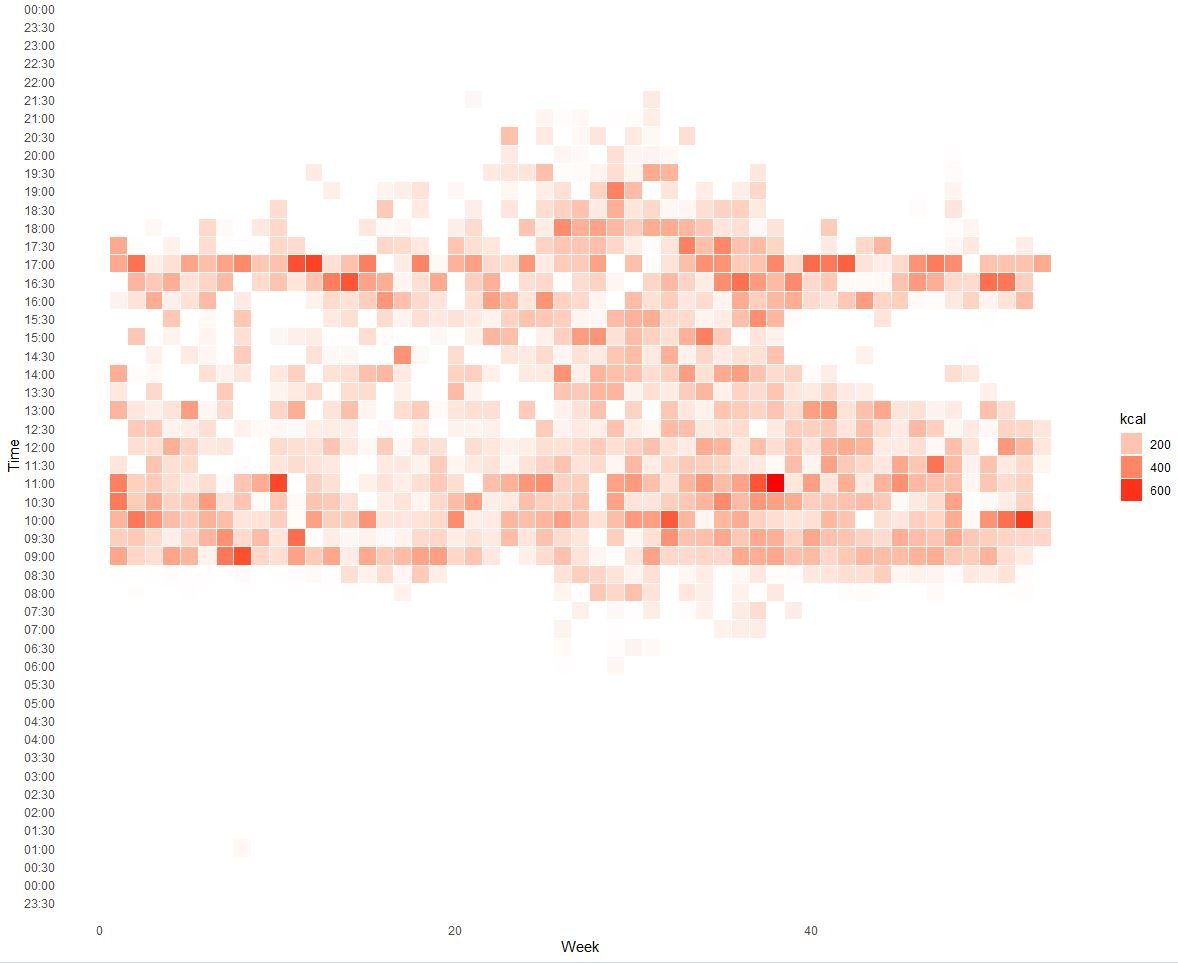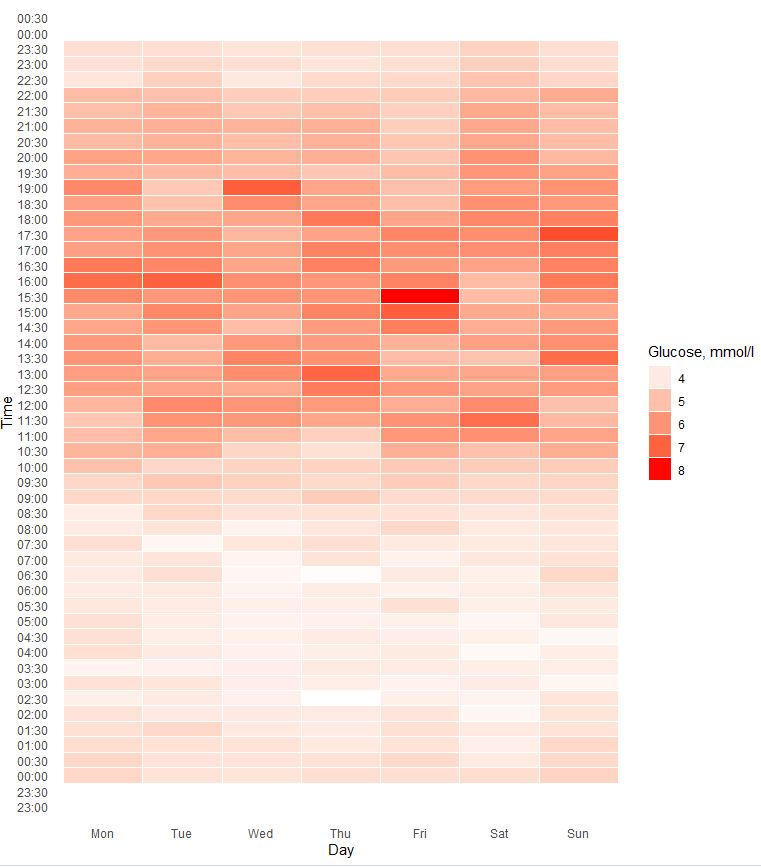Quantified Self Experiments / Data Visualization - TRE, Blood Glucose and optimal dinner time

## Time Restricted Eating

Time restricted eating (TRE) seems to have good impact on our healt.There is good explanations of scientific background in a Circadian Code book by Satchin Panda. Various studies with TRE as intervention shows promising results in keeping metabolic health in a good shape.

Since i have detailed food log for a long time, i can check what is my usual eating window.

Heatmap of calorie intake by time:X - week number, Y - time of day, Color - food intake in kcal (sum).

As we can see my usual breakfast time is around 9:00 and dinner before 17:30 which is 8.5 hours average time window and confirms my constant meal regime.

## Dailly Glucose

Lets check what is my blood glucose daily pattern looks like:40 days of data grouped by day of week

## Optimal dinner time

Even finishing dinner before 17:30 results in postprandial glucose elevation until 21:30-22:00. My usual bedtime start is 22:00-22:30. It seems i should keep my dinner before 17:30 and avoid late meals to make sure my body have enough time to deal with it before i go sleep.

## Data availability & Information

Data is available for calorie intake here and for CGM glucose (FreeStyle Libre) here

`#Calorie intake`

`library(lubridate)library(dplyr)library(plyr)library(ggplot2)df <- read.csv("https://blog.kto.to/uploads/food-kcal.csv")df <- df[!is.na(df\$`kcal`),];df\$ymd <-ymd_hms(df\$datetime)df\$date <-as.Date(df\$ymd)df\$month <- month(df\$datetime, label = TRUE)df\$year <- year(df\$datetime)df\$day <- day(df\$datetime)df\$week <- week(df\$datetime)df\$wday <- wday(df\$datetime, label = TRUE)df\$hour <- hour(df\$datetime)df\$minute <- 60*60*df\$hour + round(minute(df\$datetime)/30)*30*60df\$time <- format(as.POSIXct('1900-1-1') + df\$minute, '%H:%M:%S')hdf <- ddply(df, c( "time", "week"), summarise, kcal = sum(kcal)/7)hdf\$x <- hdf\$week#hdf\$x <- factor(hdf\$month, levels=rev(levels(hdf\$month)))hdf\$y <- as.POSIXct(strptime(hdf\$time, format = "%H:%M:%S"))hdf\$f <- hdf\$kcallibrary(scales)ggplot(hdf, aes(x, y)) + geom_tile(aes(fill = f), colour = "white", na.rm = TRUE) +  scale_fill_gradient(low = "white", high = "red") +    guides(fill=guide_legend(title="kcal")) +  scale_y_datetime(date_breaks = "30 min", date_labels = "%H:%M") +  theme_bw() + theme_minimal() +   labs(title = "Histogram of Food", x = "Week", y = "Time") +  theme(panel.grid.major = element_blank(), panel.grid.minor = element_blank())\`

`#Glucose`

`library(lubridate)library(dplyr)library(plyr)library(ggplot2)#sleep status for 30 sec periodsdf <- read.csv("https://blog.kto.to/uploads/cgm.csv") df <- df[!is.na(df\$`glucose`),];df\$ymd <-ymd_hms(df\$datetime)df\$date <-as.Date(df\$ymd)df\$month <- month(df\$datetime, label = TRUE)df\$year <- year(df\$datetime)df\$day <- day(df\$datetime)df\$week <- week(df\$datetime)df\$wday <- wday(df\$datetime, label = TRUE)df\$hour <- hour(df\$datetime)df\$minute <- 60*60*df\$hour + round(minute(df\$datetime)/30)*30*60df\$time <- format(as.POSIXct('1900-1-1') + df\$minute, '%H:%M:%S')hdf <- ddply(df, c( "time", "wday"), summarise, glucose = mean(glucose))dayLabs<-c("Mon","Tue","Wed","Thu","Fri","Sat","Sun") hdf\$x <- factor(hdf\$wday, levels=dayLabs)hdf\$y <- as.POSIXct(strptime(hdf\$time, format = "%H:%M:%S"))hdf\$f <- hdf\$glucoselibrary(scales)ggplot(hdf, aes(x, y)) + geom_tile(aes(fill = f), colour = "white", na.rm = TRUE) +  scale_fill_gradient(low = "white", high = "red") +    guides(fill=guide_legend(title="Glucose, mmol/l")) +  scale_y_datetime(date_breaks = "30 min", date_labels = "%H:%M") +  theme_bw() + theme_minimal() +   labs(title = "Histogram of Sleep", x = "Day", y = "Time") +  theme(panel.grid.major = element_blank(), panel.grid.minor = element_blank())`

#### Statistical analysis

RStudio version 1.3.959 and R version 4.0.2.